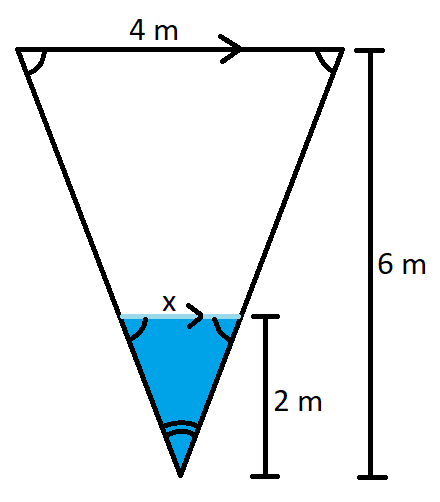top of page# SHARE

Mr Chen's anecdotes.

Search

# 3 THINGS TO NOTE IN SEC 2 MENSURATION

In primary school, mensuration is limited to simple shapes and figures. They are namely, right-angled triangles, isosceles triangles, rectangles and cuboids.

However, as we progress further in our academic journey, we are exposed to different yet commonly seen figures in daily life. They are namely, spheres, pyramids and cones.

In secondary 2 math, students are introduced to formulas of volume and surface area of such shapes. It can be an easy chapter. However, such questions are often intertwined with other chapters and it would be incredibly useful if you keep the following things in mind.

(Follow @vantage_tutor on Instagram to get video updates whenever a new article is released)

## Formulas

In order to determine the volume of a figure, one has to first know the formula for each individual shape. The easiest thing to do is to memorize the various formulas. Fortunately, at a higher level, the formula for volume and surface area of figures would be provided in the O level Math formula sheet. Alternatively, it would be given in the question's preamble.

Personally, I never saw the need to memorize the formulas apart from those concerning spheres. For the volume of any figure, it is always the product of the base area and the perpendicular height. Essentially, you're shading upwards like a color pencil to find the volume. (Consider how you eat your Nyonya kueh). The only thing to keep in mind is figures with a vertex would need to be multiplied by one-third.I used to peel the layers apart and eat the individual layers.

Back to Table of Content

## Similar Triangles

For IP2 Math, similar triangles are often tested together with mensuration. Prior to applying the formula for the volume of a figure, all the dimensions are required to be known. This unknown dimension can often be found through the application of proportions.A classic example would be a question asking to determine the volume of water in a cone. Very often, the depth of water is given, and the dimensions of the container are given clearly. However, in order to determine the volume of the water, the radius is required. The radius can be found by comparing the height of the container and the depth of water. This is because the shape of the container is similar to the shape of the water. Determine the proportion, and calculate for the radius before finally, substituting the respective values into the volume formula.

Back to Table of Content

## Pythagoras' Theorem

Methods to determine unknown dimensions are not limited to just similar triangles. In fact, when you learn Secondary 3 E Math or A Math, trigonometry would come into play as well. However, in Sec 2 Math, the other common method is through Pythagoras' Theorem.

Common examples of such questions are those involving pyramids and cones. They are often used in both the determination of surface area and volume. In the surface area of a cone, the slant height is required. A cone is consist of a right-angled triangle due to the presence of the perpendicular height. The slant height of a cone is the hypothenuse of a right-angled triangle. Hence, by application of Pythagoras' Theorem, the slant height can be determined when given the radius and perpendicular height of the figure.

Vice versa, questions may start off with slant height and radius of pyramid while asking to calculate the volume of the pyramid. Apply Pythagoras' Theorem and manipulate your terms such that the perpendicular height, h, is now the subject. Calculate for the perpendicular height and apply the volume formula to find the volume of the pyramid. (Don't forget to multiply by one-third!)

Back to Table of Content

## Mensuration Is Important

Mensuration, at the most fundamental level, is the application of math in real life. Engineers deal with volume and area all the time while you and I discuss the possible conspiracy bubble tea drink stores are engaged in.

Did we really upsize with more BBT or is it just a cup, shaped differently to look bigger?

Regardless, if you struggle with mensuration or any other topic in Sec 2 Math, you may always contact us for a trial lesson. We, at VANTAGE TUTOR, are patient and reliable. Our Math and Physics tutors have helped countless students improve their grades and achieve their academic goals in various subjects.

Besides, we can always have a conversation about how BBT is so expensive and if we are really getting scammed of our money with every upsize.

Hesitate no more. Join us and be A Class Above The Rest.